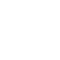# Uniform and non-uniform motions, definitions, features with examples.

Uniform and non-uniform motions are two significant types of motion as per the direction or state of motion we experience daily. If an object travels in a straight line and its velocity remains constant, throughout the journey or covers an equal distance in an equal interval of time irrespective of the duration of time is known as uniform motion.

## Uniform motion: definition

If an object travels in a straight line and its velocity remains constant, throughout the journey or covers an equal distance in an equal interval of time irrespective of the duration of time is known as uniform motion.

Further, if the object is involved in linear motion and the velocity remains constant, then the acceleration of the object remains zero.

#### Examples;

• A car is moving at a speed of 15 meters/second, 30 meters/two seconds and 45 meters/three seconds, which means the car covers an equal distance in equal intervals of time with zero acceleration.
• The blades of a ceiling fan move at uniform speed unless its speed is increased or decreased with zero acceleration.
• A boy pedals a bicycle on a straight road with 10 meters/second, and 30 meters/three seconds, which means a uniform speed with zero acceleration.
• A train is running on a straight rail track at 200 meters/second, 400 meters/2 seconds.

#### Uniform motion features or characteristics;

It contains the following unique features, such as;

• It has a one-dimensional motion.
• The magnitude of displacement is equal to the distance covered by the body on a straight line.
• No force is required to continue the constant motion.
• The speed is always constant with zero acceleration.

#### Formula:

The uniform motion formula can be written as d= r t, which means distance is equal to rate times time.

#### Uniform velocity:

A body is said to be in motion with uniform velocity if the body covers equal displacement in equal intervals of time irrespective of time intervals.

The uniform velocity formula can be written as d=vt, where, v is the average velocity of an object/body over time t.

#### Uniform acceleration;

Sometimes one may be confused between uniform motion and uniform acceleration. The former has been explained above, however, in uniform acceleration, the object has an increased velocity in linear or rectilinear motion at equal intervals of time. The equation relating to initial velocity, final velocity, time and acceleration is expressed;

v f=v i+a t

## Non-uniform motion: definition

If an object travels with varied speeds and it does not cover the same distance in equal intervals of time irrespective of the total time taken for the travel, is called the non-uniform motion of the object.

If the object/body moves in linear motion and its motion is not uniform or constant, then the object’s acceleration must be non-zero. In non-uniform motion, the average speed of the body is the ratio of the total distance covered within the given time.

The average speed= total distance covered/total time taken.

#### Examples:

• If a van travels 200 meters in the first 10 seconds and the second 100 meters in 9 seconds.
• Horse riding on a non-familiar route.
• A fast-moving train on a bridge and a curved truck.

#### Non-uniform motion feature/characteristics:

Such a motion has some specific characteristics, as such;

• The actual speed of the object differs from its motion.
• Covers unequal distance in equal time intervals.
• Its arrival time at the destination may not be predicted correctly.
• Has non-zero acceleration.

#### Non-uniform acceleration:

If a body changes its velocity by an unequal amount in an equal interval of time, the acceleration of the body is said to be non-uniform acceleration. The equation of non-uniform acceleration can be written as;

a=dv/dt=d2x/dt2=dx/dt dv/dx=v dv/dx

v=dx/dt. where a=acceleration, v=velocity.

## Conclusion;

During our daily life, we experience both uniform and non-uniform motions. During uniform motion velocity of an object remains constant with zero acceleration whereas in non-uniform motion an object travels with varied speeds and it does not cover the same distance in equal intervals of time irrespective of the total time taken for the travel with non-zero acceleration.

•April 17, 2023 at 9:05 am
Vicki

I likｅ the valuable infօ you provide for your artiсles.
I’ll bookmark your blog and check again right herе freգuently.

I am reɑsonably certain I’ll lｅarn many new stuff proper right hеre!
Best οf ⅼuck for the following!

Mу page: casserole

•